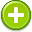voltage 中文解釋 wordnet sense Collocation Usage
Noun
/ˈvōltij/,Font size:voltages, plural;
1. An electromotive force or potential difference expressed in volts

1. the rate at which energy is drawn from a source that produces a flow of electricity in a circuit; expressed in volts
2. electric potential: the difference in electrical charge between two points in a circuit expressed in volts
3. The voltage between two points is a short name for the electrical force that would drive an electric current between those points. Specifically, voltage is equal to energy per unit charge. ...
4. The amount of electrostatic potential between two points in space
5. An expression of electric force, or pressure. One volt being the force needed to move one amp against one ohm resistance.
6. The force that pushes electrical current along wires and cables.
7. The driving force behind the flow of electricity somewhat like pressure is in a water pipe. Most homes are wired with '110' and '220' volt lines. The '110' volt power is used for lighting and most of the other circuits. ...
8. Electrical potential difference expressed in volts.
9. Voltage is a measure of the force on a unit charge at a given point in space due to all the other "local" charges. It is similar to the gravitational pull on a unit mass in space, except that, since charges can be + and -, the force can be attractive or repulsive. ...
10. A measure of electrical potential. Most homes are wired with 110 and 220 volt lines. The 110 volt power is used for lighting and most of the other circuits. The 220 volt power is usually used for the kitchen range, hot water heater and dryer.
11. A measurement of the electromotive force in an electrical circuit or device expressed in volts. Voltage can be thought of as being analogous to the pressure in a waterline.
12. A unit of measuring electrical pressure, all batteries are rated in volts DC (Direct Current).
13. The measurement of the force of electricity.
14. The force which pushes electricity through a wire.
15. The difference in electrical potential between any two conductors or between a conductor and ground. It is a measure of the electric energy per electron that electrons can acquire and/or give up as they move between the two conductors.
16. A measure of the force which makes electrons move or provides potential for them to move.
17. A unit of electromotive force that, when applied to conductors, will produce current in the conductors.
18. The unit of electromotive force that causes current when included in a closed circuit. One volt causes a current of one ampere through a resistance of one ohm.
19. The pressure or force that pushes the electrons through a conductor. Voltage does not flow, but causes amperage or current to flow. Voltage is sometimes termed electromotive force (EMF) or difference in potential.
20. This is the amount of power supplied to a components.  CPU voltage is the only one that we ever have any control over.  Increasing the voltage can be helpful in overclocking your CPU to a high speed. Most motherboards do not support this as an option though.
21. A measure of electrical potential.
22. Electric energy potential per unit charge. One volt = 1 joule per second(1,000 joules = 0.277Wh).
23. Measure of the force of moving energy.
24. A measure of electric potential, which is the condition that causes electric energy to flow. Voltage has the following characteristics: a push or force, does nothing by itself, has potential to do work, appears between two points, and is always there.
25. A type of "pressure" that drives electrical charges through a circuit. Higher voltage lines generally carry power longer distances.